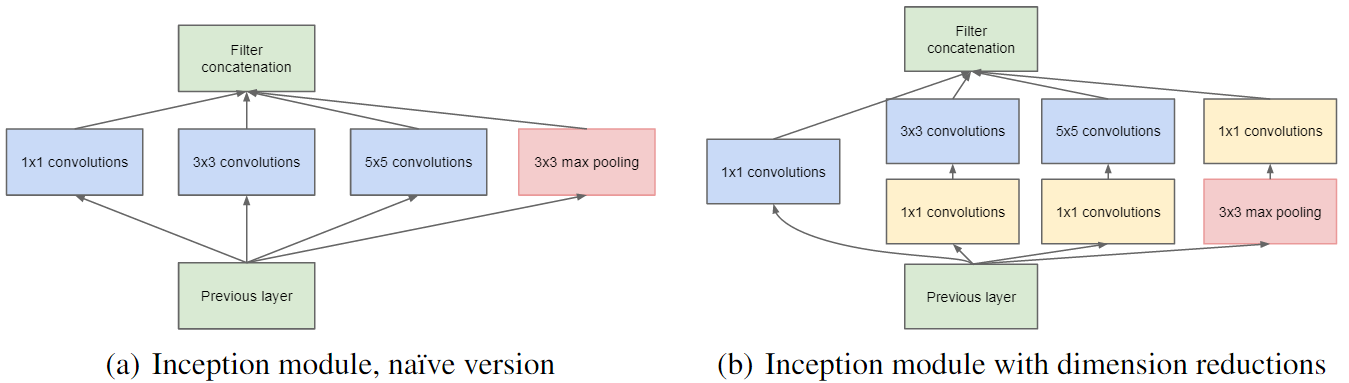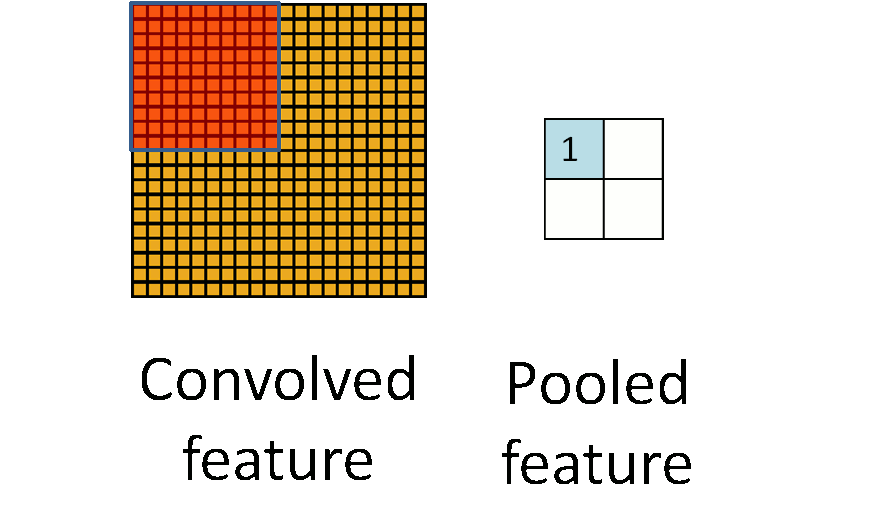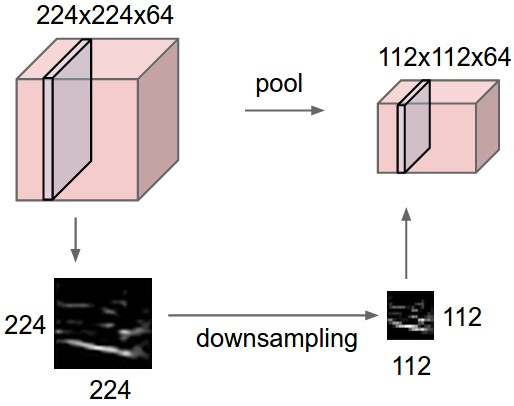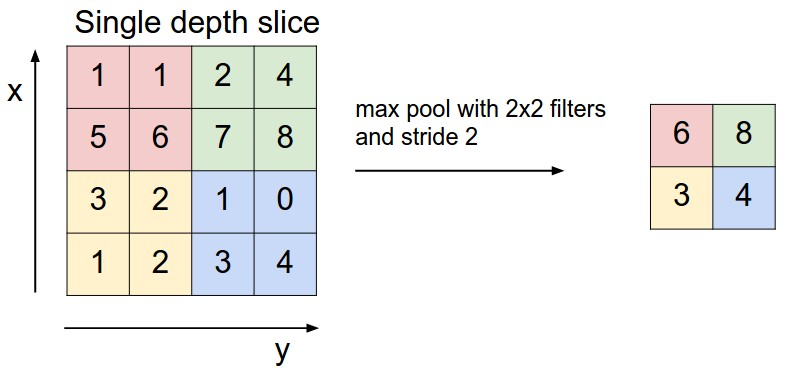# Layers of a Convolutional Neural Network

Convolutional neural networks are built by concatenating individual blocks that achieve different tasks. These building blocks are often referred to as the layers in a convolutional neural network. In this section, some of the most common types of these layers will be explained in terms of their structure, functionality, benefits and drawbacks.

This section is an excerpt from Convolutional Neural Networks

## Convolutional Layer

The main task of the convolutional layer is to detect local conjunctions of features from the previous layer and mapping their appearance to a feature map. As a result of convolution in neuronal networks, the image is split into perceptrons, creating local receptive fields and finally compressing the perceptrons in feature maps of size  $//$. Thus, this map stores the information where the feature occurs in the image and how well it corresponds to the filter. Hence, each filter is trained spatial in regard to the position in the volume it is applied to.

In each layer, there is a bank of   $//$ filters. The number of how many filters are applied in one stage is equivalent to the depth of the volume of output feature maps. Each filter detects a particular feature at every location on the input. The output  $//$  of layer  $//$ consists of  $//$ feature maps of size  $//$. The  $//$ feature map, denoted  $//$, is computed as

 (1)

where  $//$ is a bias matrix and   $//$ is the filter of size  $//$ connecting the  $//$ feature map in layer  $//$ with  $//$ feature map in layer.

The result of staging these convolutional layers in conjunction with the following layers is that the information of the image is classified like in vision. That means that the pixels are assembled into edglets, edglets into motifs, motifs into parts, parts into objects, and objects into scenes.

Original version of the page created by Simon Pöcheim can be found here.

## Non-Linearity Layer

A non-linearity layer in a convolutional neural network consists of an activation function that takes the feature map generated by the convolutional layer and creates the activation map as its output. The activation function is an element-wise operation over the input volume and therefore the dimensions of the input and the output are identical.

In other words; let layer $//$ be a non-linearity layer, it takes the feature volume $//$ from a convolutional layer $//$ and generates the activation volume $//$:

with,

This can also be interpreted as $//$ number of 2-dimensional feature maps (generated by $//$ number of filters in convolutional layer $//$) each with size $//$.

In some publications(2) a gain koefficient is added to the activation function in order to cope up with the vanishing gradient problem:

Similar to multilayer perceptrons, the activation function is generally implemented as logistic (sigmoid) or hyperbolic tangent functions. However, more recent research suggests rectified linear units (ReLUs) are advantageous over the traditional activation functions particularly in convolutional neural networks (3).

It is noteworthy that, although this wiki seperates the non-linearity layer from convolutional layer, it is not uncommon to see a combination of the non-linearity layer with the convolutional layer in the literature. Some of the works use different notations for their layer and architecture description. In some publications(2) of Yann LeCun for example, the combined layer is named a filter bank layer and represented as  $//$ denoting a convolutional layer ( $//$) with a hyperbolic tangent activation function ( $//$) and gain coefficients ( $//$) .Logistic (sigmoid) and hyperbolic tangent functions are commonly used activation functions in convolutional neural networks.

## Rectification Layer

A rectification layer in a convolutional neural network performs element-wise absolute value operation on the input volume (generally the activation volume). Let layer $//$ be a rectification layer, it takes the activation volume $//$ from a non-linearity layer $//$ and generates the rectified activation volume $//$:

Similar to the non-linearity layer, the element-wise operation properties do not change the size of the input volume and therefore, these operations can be (and in many cases(1) including AlexNet(4) and GoogLeNet(5) are) merged into a single layer:

Regardless of the general simplicity of the operation, it plays a key role in the performance of the convolutional neural network by eliminating cancellation effects in subsequent layers. Particularly when an average pooling method is utilized, the negative values within the activation volume are prone to cancel out the positive activations, degrading the accuracy of the network significantly. Therefore, the rectification is named as a "crucial component" (2).

## Rectified Linear Units (ReLU)

The rectified linear units (ReLUs) are a special implementation that combines non-linearity and rectification layers in convolutional neural networks. A rectified linear unit (i.e. thresholding at zero) is a piecewise linear function defined as:

The rectified linear units come with three significant advantages in convolutional neural networks compared to the traditional logistic or hyperbolic tangent activation functions:

• Rectified linear units propagate the gradient efficiently and therefore reduce the likelihood of a vanishing gradient problem that is common in deep neural architectures.
• Rectified linear units threshold negative values to zero, and therefore solve the cancellation problem as well as result in a much more sparse activation volume at its output. The sparsity is useful for multiple reasons but mainly provides robustness to small changes in input such as noise (6).
• Rectified linear units consist of only simple operations in terms of computation (mainly comparisons) and therefore much more efficient to implement in convolutional neural networks.

As a result of its advantages and performance, most of the recent architectures of convolutional neural networks utilize only rectified linear unit layers (or its derivatives such as noisy or leaky ReLUs) as their non-linearity layers instead of traditional non-linearity and rectification layers.

In works such as AlexNet(4) rectified linear units are shown to operate six times faster then hyperbolic tangent non-linearities while reaching 25% error rate on CIFAR-10 dataset(7). More recently, utilization of an advanced derivative Parametric Rectified Linear Units (PReLU) allowed convolutional networks to surpass human-level performance in ImageNet database (8). More information on the advantages of the rectified linear units in convolutional neural networks can be found in (3).Rectified linear units are becoming more popular among their counterparts due to their simplicity and multiple advantages.

## Pooling Layer

The pooling or downsampling layer is responsible for reducing the spacial size of the activation maps. In general, they are used after multiple stages of other layers (i.e. convolutional and non-linearity layers) in order to reduce the computational requirements progressively through the network as well as minimizing the likelihood of overfitting.

The pooling layer $//$ has two hyperparameters, the spatial extent of the filter  $//$ and the stride $//$. It takes an input volume of size $//$ and provides an output volume of size $//$ where;

The key concept of the pooling layer is to provide translational invariance since particularly in image recognition tasks, the feature detection is more important compared to the feature's exact location. Therefore the pooling operation aims to preserve the detected features in a smaller representation and does so, by discarding less significant data at the cost of spatial resolution.

The pooling layer operates by defining a window of size $//$ and reducing the data within this window to a single value. The window is moved by $//$ positions after each operation similarly to the convolutional layer and the reduction is repeated at each position of the window until the entire activation volume is spatially reduced.

It is noteworthy that the window for pooling layers does not have to be a square and can be parametrised with $//$ and $//$ resulting in a rectangle of size $//$. However, this is extremely uncommon and is therefore left out of the notation is most of the publications, including this wiki.

The most common methods for reduction are max pooling and average pooling. Max pooling operates by finding the highest value within the window region and discarding the rest of the values. Average pooling on the other hand uses the mean of the values within the region instead.

Max pooling has demonstrated faster convergence and better performance in comparison to the average pooling and other variants such as  $//$-norm pooling (9). Thus, recent work generally trends towards max pooling or similar variants.

Apart from the reduction method, the hyperparameter selections determine whether the pooling windows overlap or not. In case $// S^{(l)}\end{array} //]]>$ the pooling windows overlap on top of each other, filtering some of the data points multiple times. Depending on this condition, a pooling layer is named overlapping or non-overlapping pooling.

Even though the overlapping window method has been used with success to some extent and some of the research(4) suggests that it can reduce the chance of overfitting on certain datasets, the larger spatial size $//$ in pooling layers is generally considered destructive (13). Therefore, the most commonly used pooling parameters are $//$ which is non-overlapping.

The selection of $//$ is the smallest feasible integer sized filter, however, it still discards 75% of the data.This aggressive reduction executed by pooling layers can limit the depth of a network and ultimately limit the performance. This problem has pushed the research to find other methods to improve or replace the pooling layer.

One approach is to use smaller "fractional" filters(10) instead of common ones. Another option is to remove pooling layers completely and simply perform the reduction by increasing the stride in convolutional layers (11). These solutions still remain as active research topics.

It is important to note that some of the cutting edge architectures such as GoogLeNet's Inception Modules also feature $//$ convolutions to reduce the dimensions of the activation volume before a $//$ or  $//$ convolutional layer. These are not considered as pooling layers since the reduction is not happening stritctly spatially but along the depth axis. However, the architecture of the GoogLeNet still features max and average pooling layers between the inception modules apart from its $//$ convolutions. (5)GoogLeNet's Inception Module architecture depicting the underlying $//$ convolutions. (Image source (5))An animation depicting a  $//$ input being reduced to  $//$ output using  $//$ and  $//$ non-overlapping pooling operation. (Image source (12))Pooling is performed spatially on each 2-dimensional map within the volume. As a result the depth of the output volume is the same as the input volume.(Image source (13))Non-overlapping max pooling on a 2-dimensional $//$ input. 75% of the data is discarded in the resulting  $//$ output.(Image source (12))

## Fully Connected Layer

The fully connected layers in a convolutional network are practically a multilayer perceptron (generally a two or three layer MLP) that aims to map the $//$ activation volume from the combination of previous different layers into a class probability distribution. Thus, the output layer of the multilayer perceptron will have $//$ outputs, i.e. output neurons where  $//$ denotes the number of layers in the multilayer perceptron.

The key difference from a standard multilayer perceptron is the input layer where instead of a vector, an activation volume is taken as the input. As a result the fully connected layer is defined as:

If $//$ is a fully connected layer;

otherwise;A three layer fully connected multilayer perceptron structure that is identical to a fully connected layer in convolutional neural networks with only difference being the input layer.(Image source)

The goal of the complete fully connected structure is to tune the weight parameters  $//$ or  $//$ to create a stochastic likelihood representation of each class based on the activation maps generated by the concatenation of convolutional, non-linearity, rectification and pooling layers. Individual fully connected layers operate identically to the layers of the multilayer perceptron with the only exception being the input layer.

It is noteworthy that the function  $//$ once again represents the non-linearity, however, in a fully connected structure the non-linearity is built within the neurons and is not a seperate layer.

As a contradiction, according to Yann LeCun, there are no fully connected layers in a convolutional neural network and fully connected layers are in fact convolutional layers with a  $//$ convolution kernels (14). This is indeed true and a fully connected structure can be realized with convolutional layers which is becoming the rising trend in the research.

As an example; the AlexNet(4) generates an activation volume of  $//$ prior to its fully connected layers dimensioned with  $//$$//$$//$ neurons respectively. The first layer can be replaced with a convolutional layer consisting of  $//$ filters, each with a size of  $//$ resulting in a  $//$ output, which in fact is only a 1-dimensional vector of size  $//$.The architecture of AlexNet also depicting its dimensions including the fully connected structure as its last three layers.(Image source (4))

Subsequently, the second layer can be replaced with a convolutional layer consisting of  $//$ filters again, each with a size of  $//$ resulting in a  $//$ output once again. Ultimately, the output layer can be replaced with a convolutional layer consisting of  $//$ filters, each with a size of  $//$ resulting in a  $//$ output, which yields the classification result of the image among  $//$ classes (13).

  http://ufldl.stanford.edu/tutorial/supervised/Pooling/ (Last visited: 21.01.2017)

  http://cs231n.github.io/convolutional-networks/#fc/ (Last visited: 21.01.2017)

• No labels

1. All the comments are SUGGESTIONS and are obviously highly subjective!

• Try to incorporate links to other pages (e.g., multi-layer perceptron, CNNs...)
• Check for redundant spaces
• Give numbers to all figures and formulas and refer to them by number (e.g., Equation 1). Avoid "below" etc.
• Maybe provide a reference to the CIFAR-10 database
• Introduce abbreviations when you first use them (e.g., MLP). I would then always use them to save space (FCL, MLP, ...)

• Normally you try to avoid shortenings like "it's" "that's" "won't" but use "it is" that is" "will not"

Corrections:

•  image and mapping their appearance to a feature map

•  image and map their appearance to a feature map

• neuronal network

• neural network

• can be (and in many cases(1) including AlexNet(4) and GoogLeNet(5) is)

• can be (and in many cases(1) including AlexNet(4) and GoogLeNet(5) are)

• Even though the general simplicity of the operation, it plays a key role in the performance

• Regardless of the general simplicity of the operation, it plays a key role in the performance

• Therefore the rectification is named as a "crucial component" (2).

• Therefore, the rectification is named as a "crucial component" (2).
•  and therefore solves the cancellation problem as well as resulting
•  and therefore solve the cancellation problem as well as result
• (mainly comparison)
• (mainly comparisons)
• convolutional neural networks feature only rectified linear unit layers
• convolutional neural networks feature only use rectified linear unit layers
• window for pooling layer does
• window for pooling layers does
• However this is extremely uncommon and is therefore left out of the notation is most of the works, including this wiki.
• However, this is extremely uncommon and is therefore left out of the notation in most of the publications, including this wiki.
• Even though overlapping window method has been used with success
• Even though the overlapping window method has been used with success
• the larger spatial size  F(l) in pooling layers are generally considered
• the larger spatial size  F(l) in pooling layers is generally considered
• Therefore the most commonly used pooling parameters are F(l)=2,S(l)=2 which is non-overlapping.
• Therefore, the most commonly used pooling parameters are F(l)=2,S(l)=2 which are non-overlapping.
• however it still discards 75% of the data
• however, it still discards 75% of the data
• not happening stritctly spatially but in depth axis
• not happening strictly spatially but along the depth axis
• outputs i.e. output neurons where i  denotes
• outputs, i.e., output neurons where i  denotes        (I know the commas look weird, but this is how it is in American English)
• from a standart multilayer perceptron
• from a standard multilayer perceptron
• non-linearity, however in a fully connected structure, the non-linearity is built within the neurons and is not a seperate layer
• non-linearity, however, in a fully connected structure the non-linearity is built within the neurons and is not a separate layer
• As a contradiction, according to Yann LeCun there are no fully connected layers
• As a contradiction, according to Yann LeCun, there are no fully connected layers
• volume of 512×7×7  512×7×7 before its fully connected
• volume of 512×7×7  512×7×7 prior to its fully connected
• Subsequently, second layer can be replaced
• Subsequently, the second layer can be replaced

Confusion:

•  Individual fully connected layers function identically (???) to the layers of the multilayer perceptron with the only exception being the input layer.      (a verb is missing here)

Final remark:

• I liked your contribution a lot. Mathematical detail but still easy to follow. Sometimes maybe too much emphasis und the rigorous notation for the introductory part.
• Sorry that it looks like I ripped your stuff apart. Most mistakes are just on commas, but since there is no "Review" function as in Word, I have to copy all the sentences to make it clear
2. Thank you very much for the amazing contribution in such short time. I agree with almost all of your comments and they will be fixed, some points though i am unsure which way would work better so i would like to share them with you:

• Give numbers to all figures and formulas and refer to them by number (e.g., Equation 1). Avoid "below" etc: In a scientific paper or thesis writing i would 100% agree with you. I also agree that wording such as "below" or "above" should be avoided even in a wiki, hence i formulated them as a part of a sentence or paragraph following the general Wikipedia page style. I am unsure which would be "better" or "correct" though.

• Introduce abbreviations when you first use them (e.g., MLP). I would then always use them to save space (FCL, MLP, ...): In a straight-forward reading this is indeed helpful but once again I am unsure which would be more readable in a wiki since most wiki pages are not read from start but read partially to get informed about the relevant section. Particularly in a the "Layers of CNN" context i am unsure if using abbreviations after defining them once would be helpful.

• Normally you try to avoid shortenings like "it's" "that's" "won't" but use "it is" that is" "will not": Could not find any in my part except from the direct quotation from Simon's page (which will be updated as soon as his final version is ready).

Confusion:

•  Individual fully connected layers function identically (???) to the layers of the multilayer perceptron with the only exception being the input layer.      (a verb is missing here): "Function" is the verb in that sentence not the name, as in "to function". I will switch the wording to "operate" in order to avoid confusion.

Everything else i agree and now have on my to do list. Again thank your very much for such a thorough and fast review. The largest problem is that now i'm under pressure that my reviews for other people will not be even half as thorough as yours.

As a last remark, when you select a text on viewing mode (i.e., not editing mode) a small message bubble appears which you can use to add inline comment to the page which i believe is extremely useful for reviewing.

3. Hi, first of all, it is really hard to find some suggestions as the second reviewer!Regarding the language It sounds very good for me. I didn´t find any passage which I could correct or misunderstand.

The only thing I would suggest on your article is, that you could mention that there exist more Layers than all the Layers you mentioned. Like for example, DropOut Layer or BatchNormalization Layers. This is just a suggestion, but in my oppinion this will help beginners. They will see that this article is about the common layers but there will be more layers existing in common toolkits. You can also link these two other layers to the sections in the advanced level.

All in all a very good article!